Home Practice
For learners and parents For teachers and schools
Textbooks
Full catalogue
Pricing SupportLog in

We think you are located in United States. Is this correct?

# Chapter 8: Euclidean geometry

• Sketches are valuable and important tools. Encourage learners to draw accurate diagrams to solve problems.
• It is important to stress to learners that proportion gives no indication of actual length. It only indicates the ratio between lengths.
• To prove triangles are similar, we need to show that two angles (AAA) are equal OR three sides in proportion (SSS).
• Theorems are examinable and are often asked in examinations. It is also important that learners remember the correct construction required for each proof.
• Notation - emphasize to learners the importance of the correct ordering of letters, as this indicates which angles are equal and which sides are in the same proportion.
• If a length has to be calculated from a proportion, it helps to re-write the proportion with the unknown length in the top left position.

## 8.1 Revision (EMCHY)

temp text

### Types of triangles (EMCHZ)

 Name Diagram Properties Scalene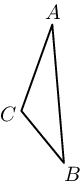All sides and angles are different. Isosceles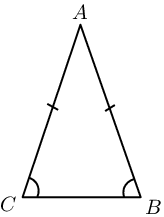Two sides are equal in length. The angles opposite the equal sides are also equal. Equilateral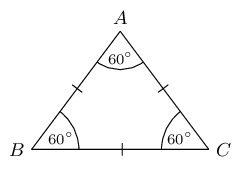All three sides are equal in length and all three angles are equal. Acute-angled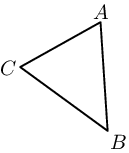Each of the three interior angles is less than $$\text{90}$$°. Obtuse-angled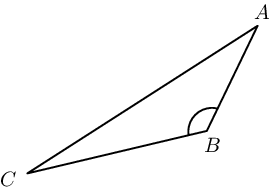One interior angle is greater than $$\text{90}$$°. Right-angled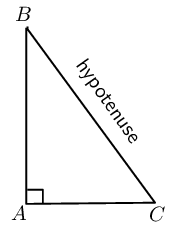One interior angle is $$\text{90}$$°.

### Congruent triangles (EMCJ2)

 Condition Diagram SSS (side, side, side)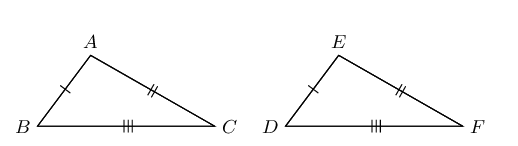$$\triangle ABC \equiv \triangle EDF$$ SAS (side, incl. angle, side)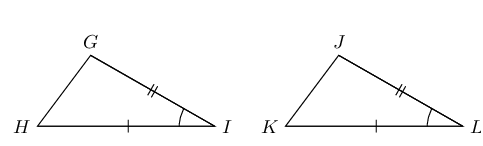$$\triangle GHI \equiv \triangle JKL$$ AAS (angle, angle, side)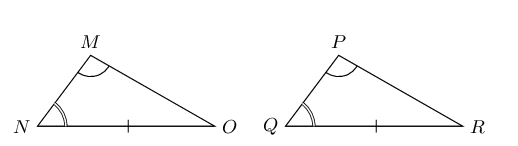$$\triangle MNO \equiv \triangle PQR$$ RHS ($$\text{90}$$°, hypotenuse, side)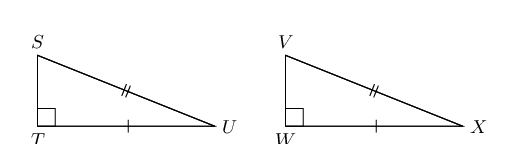$$\triangle STU \equiv \triangle VWX$$

### Similar triangles (EMCJ3)

 Condition Diagram AAA (angle, angle, angle)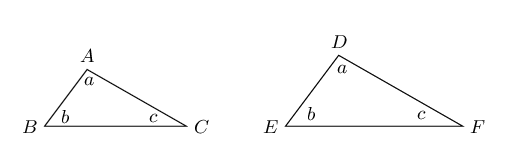$$\hat{A} = \hat{D}, \enspace \hat{B} = \hat{E}, \enspace \hat{C} = \hat{F}$$ $$\therefore \triangle ABC \enspace ||| \enspace \triangle DEF$$ SSS (sides in prop.)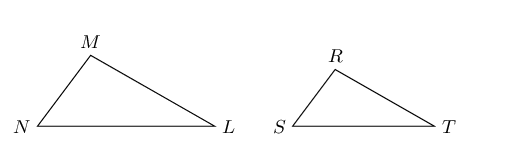$$\frac{MN}{RS} = \frac{ML}{RT} = \frac{NL}{ST}$$ $$\therefore \triangle MNL \enspace ||| \enspace \triangle RST$$

### Circle geometry (EMCJ4)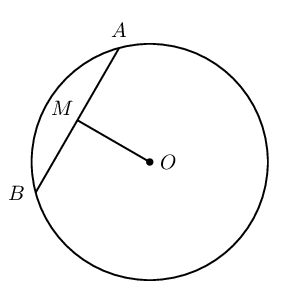If $$O$$ is the centre and $$OM \perp AB$$, then $$AM = MB$$. If $$O$$ is the centre and $$AM = MB$$, then $$A\hat{M}O = B\hat{M}O = \text{90}°$$. If $$AM = MB$$ and $$OM \perp AB$$, then $$\Rightarrow MO$$ passes through centre $$O$$.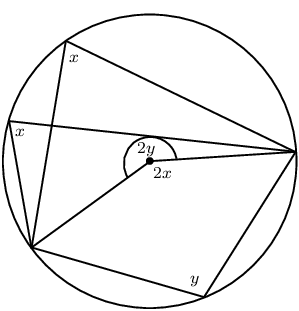If an arc subtends an angle at the centre of a circle and at the circumference, then the angle at the centre is twice the size of the angle at the circumference.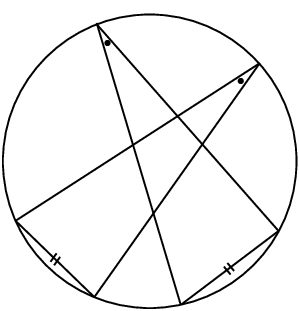Angles at the circumference subtended by arcs of equal length (or by the same arc) are equal.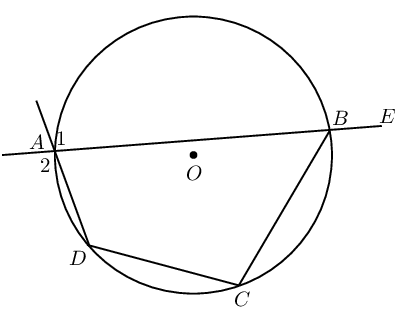If the four sides of a quadrilateral $$ABCD$$ are the chords of a circle with centre $$O$$, then: $$\hat{A} + \hat{C} = \text{180}°$$ Reason: (opp. $$\angle$$s cyclic quad. supp.) $$\hat{B} + \hat{D} = \text{180}°$$ Reason: (opp. $$\angle$$s cyclic quad. supp.) $$E\hat{B}C = \hat{D}$$ Reason: (ext. $$\angle$$ cyclic quad. = int. opp $$\angle$$) $$\hat{A}_1 = \hat{A}_2 = \hat{C}$$ Reason: (vert. opp. $$\angle$$s, ext. $$\angle$$ cyclic quad.)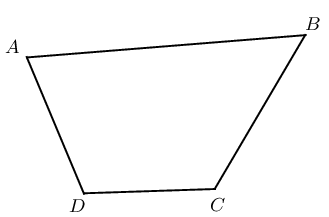If $$\hat{A} + \hat{C} = \text{180}°$$ or $$\hat{B} + \hat{D} = \text{90}´$$, then $$ABCD$$ is a cyclic quadrilateral.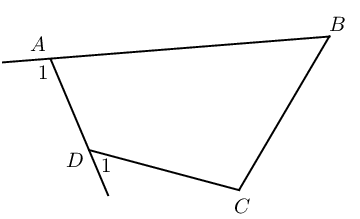If $$\hat{A}_1 = \hat{C}$$ or $$\hat{D}_1 = \hat{B}$$, then $$ABCD$$ is a cyclic quadrilateral.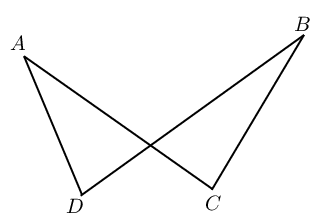If $$\hat{A} = \hat{B}$$ or $$\hat{C} = \hat{D}$$, then $$ABCD$$ is a cyclic quadrilateral.

### Tangents to a circle (EMCJ6)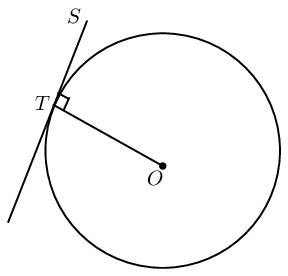A tangent is perpendicular to the radius ($$OT \perp ST$$), drawn to the point of contact with the circle.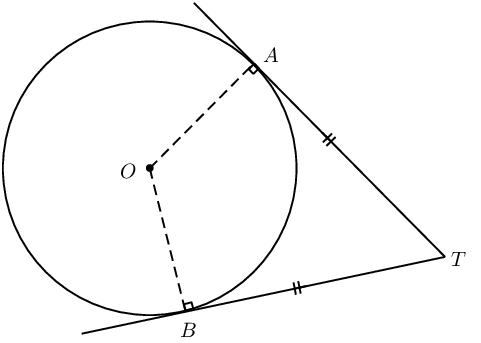If $$AT$$ and $$BT$$ are tangents to a circle with centre $$O$$, then: $$OA \perp AT$$ (tangent $$\perp$$ radius) $$OB \perp BT$$ (tangent $$\perp$$ radius) $$TA = TB$$ (tangents from same point are equal)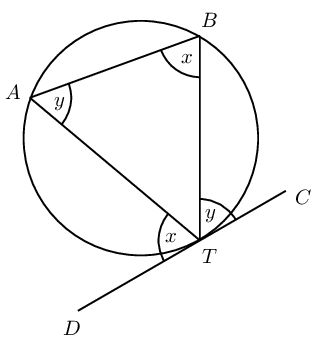If $$DC$$ is a tangent, then $$D\hat{T}A = T\hat{B}A$$ and $$C\hat{T}B = T\hat{A}B$$. If $$D\hat{T}A = T\hat{B}A$$ or $$C\hat{T}B = T\hat{A}B$$, then $$DC$$ is a tangent touching at $$T$$.

### The mid-point theorem (EMCJ7)

The line joining the mid-points of two sides of a triangle is parallel to the third side and equal to half the length of the third side.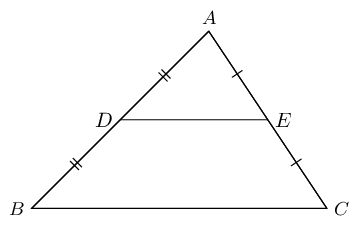Given: $$AD = DB$$ and $$AE = EC$$, we can conclude that $$DE \parallel BC$$ and $$DE = \frac{1}{2}BC$$.

## Revision

Textbook Exercise 8.1

$$MO \parallel NP$$ in a circle with centre $$O$$. $$M\hat{O}N = \text{60}°$$ and $$O\hat{M}P = z$$. Calculate the value of $$z$$, giving reasons.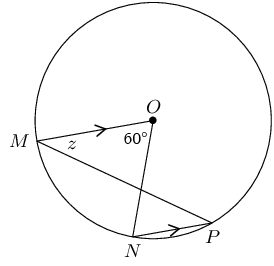$\begin{array}{rll} \hat{P} &= \frac{1}{2} M\hat{O}N & (\angle \text{ at centre = twice } \angle \text{ at circumference})\\ &= \text{30}° & \\ \therefore z &= \text{30}° &(\text{alt. } \angle\text{s, } MO \parallel NP) \end{array}$

$$O$$ is the centre of the circle with $$OC = \text{5}\text{ cm}$$ and chord $$BC = \text{8}\text{ cm}$$.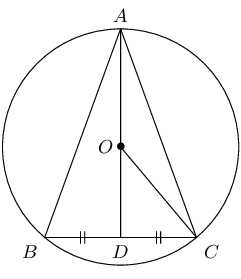Determine the lengths of:

$$OD$$
$\begin{array}{rll} \text{In } \triangle ODC, \quad OC^2 &= OD^2 + DC^2 &(\text{Pythagoras})\\ 5^2 &= OD^2 + 4^2 & \\ \therefore OD &= \text{3}\text{ cm} & \end{array}$
$$AD$$
$\begin{array}{rll} AO &= \text{5}\text{ cm} &\text{(radius)} \\ AD &= AO + OD & \\ &= \text{5} + \text{3} & \\ \therefore AD &= \text{8}\text{ cm} & \\ \end{array}$
$$AB$$
$\begin{array}{rll} \text{In } \triangle ABD, \quad AB^2 &= BD^2 + AD^2 &(\text{Pythagoras}) \\ AB^2 &= 4^2 + 8^2 & \\ AB &= \sqrt{80} & \\ \therefore AB &= 4\sqrt{5}\text{cm} & \end{array}$

$$PQ$$ is a diameter of the circle with centre $$O$$. $$SQ$$ bisects $$P\hat{Q}R$$ and $$P\hat{Q}S = a$$.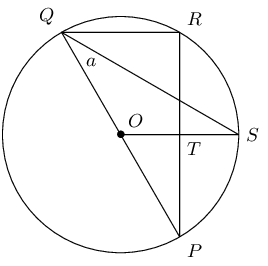Write down two other angles that are also equal to $$a$$.

$\begin{array}{rll} R\hat{Q}S &= a & (\text{given } SQ \text{ bisects } P\hat{Q}R) \\ OQ &= OS & (\text{equal radii}) \\ \therefore O\hat{Q}S &= O\hat{S}Q = a & (\text{isosceles } \triangle OQS) \end{array}$

Calculate $$P\hat{O}S$$ in terms of $$a$$, giving reasons.

$\begin{array}{rll} P\hat{O}S &= \text{2}\times P\hat{Q}S&(\angle \text{s at centre and circumference on same chord}) \\ &= \text{2}a & \end{array}$

Prove that $$OS$$ is a perpendicular bisector of $$PR$$.

$\begin{array}{rll} R\hat{Q}S &= Q\hat{S}O = a & (\text{proven}) \\ \therefore QR &\parallel OS & (\text{alt. } \angle \text{s equal}) \\ \therefore \hat{R} &= R\hat{T}S & (\text{alt. } \angle \text{s, } QR \parallel OS)\\ &= \text{90}° &(\hat{R} = \angle \text{ in semi-circle})\\ \therefore PT &= TR & (\perp \text{from centre bisects chord})\\ \therefore OS &\text{ perp. bisector of } PR & \end{array}$

$$BD$$ is a diameter of the circle with centre $$O$$. $$AB = AD$$ and $$O\hat{C}D = \text{35}°$$.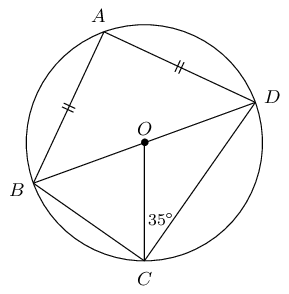Calculate the value of the following angles, giving reasons:

$$O\hat{D}C$$
$\begin{array}{rll} OC &= OD &(\text{equal radii }) \\ \therefore O\hat{D}C &= \text{35}°&(\text{isosceles } \triangle OCD) \end{array}$
$$C\hat{O}D$$
$\begin{array}{rll} C\hat{O}D &= \text{180}° - \left( \text{35}° + \text{35}° \right)&(\text{sum } \angle\text{s } \triangle = \text{180}°) \\ &=\text{110}° & \end{array}$
$$C\hat{B}D$$
$\begin{array}{rll} C\hat{B}D &= \frac{1}{2} C\hat{O}D&(\angle\text{ at centre } = 2\angle\text{ at circum. } )\\ &= \text{55}° & \end{array}$
$$B\hat{A}D$$
$\begin{array}{rll} B\hat{A}D &= \text{90}° & (\angle\text{ in semi-circle}) \end{array}$
$$A\hat{D}B$$
$\begin{array}{rll} A\hat{D}B &= A\hat{B}D &(\text{isosceles } \triangle ABD) \\ \therefore A\hat{D}B &= \frac{\text{180}°-\text{90}°}{2}&(\text{sum } \angle\text{s in } \triangle = \text{180}°)\\ &= \text{45}° & \end{array}$

$$O$$ is the centre of the circle with diameter $$AB$$. $$CD \perp AB$$ at $$P$$ and chord $$DE$$ intersects $$AB$$ at $$F$$.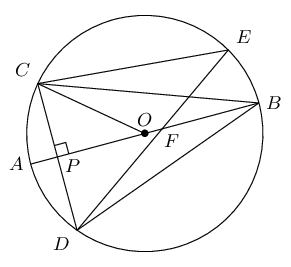Prove the following:

$$C\hat{B}P = D\hat{B}P$$
$\begin{array}{rll} \text{In } \triangle CBP &\text{ and } \triangle DBP\text{:}& \\ CP &= DP& (OP \perp CD)\\ C\hat{P}B &= D\hat{P}B = \text{90}° & (\text{given}) \\ BP &= BP & (\text{common})\\ \therefore \triangle CBP &\equiv \triangle DBP &(\text{SAS} ) \\ \therefore C\hat{B}P &= D\hat{B}P & (\triangle CBP \equiv \triangle DBP) \end{array}\\$
$$C\hat{E}D = 2 C\hat{B}A$$
$\begin{array}{rll} C\hat{E}D &= C\hat{B}D& (\angle\text{s on chord } CD)\\ \text{But } C\hat{B}A &= D\hat{B}A & (\triangle CBP \equiv \triangle DBP)\\ \therefore C\hat{E}D &= 2 C\hat{B}A \end{array}$
$$A\hat{B}D = \frac{1}{2} C\hat{O}A$$
$\begin{array}{rll} D\hat{B}A &= C\hat{B}A & (\triangle CBP \equiv \triangle DBP)\\ C\hat{B}A &= \frac{1}{2} C\hat{O}A &(\angle\text{ at centre } = 2\angle\text{ at circum. } )\\ \therefore A\hat{B}D &= \frac{1}{2} C\hat{O}A & \end{array}$

$$QP$$ in the circle with centre $$O$$ is extended to $$T$$ so that $$PR = PT$$. Express $$m$$ in terms of $$n$$.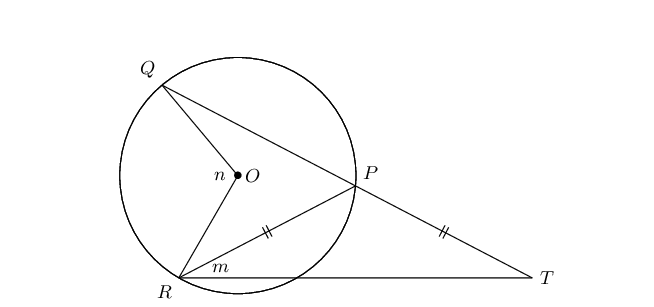$\begin{array}{rll} \hat{T} &= m &(PT = PR)\\ \therefore Q\hat{P}R &= \text{2}m &(\text{ext. } \angle \triangle = \text{ sum int. } \angle\text{s})\\ \therefore n &= \text{2}(\text{2}m)& (\angle\text{s at centre and circumference on } QR)\\ n &= 4m & \\ \therefore m &= \frac{1}{4}n & \end{array}$

In the circle with centre $$O$$, $$OR \perp QP$$, $$QP = \text{30}\text{ mm}$$ and $$RS = \text{9}\text{ mm}$$. Determine the length of $$y$$.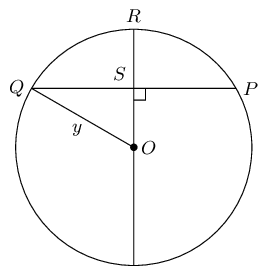$\begin{array}{rll} \text{In } &\triangle QOS, & \\ QP &= 30 & ( \text{given}) \\ QS &= \frac{1}{2}QP & (\perp \text{ from centre bisects chord}) \\ \therefore QS &= 15 & \\ QO^2 &= OS^2 + QS^2 & ( \text{Pythagoras}) \\ y^2 &= (y-9)^2 + 15^2 & \\ y^2 &= y^2 - 18y + 81 + 225 & \\ \therefore 18y &= 306 & \\ \therefore y &= \text{17}\text{ mm} & \end{array}$

$$PQ$$ is a diameter of the circle with centre $$O$$. $$QP$$ is extended to $$A$$ and $$AC$$ is a tangent to the circle. $$BA \perp AQ$$ and $$BCQ$$ is a straight line.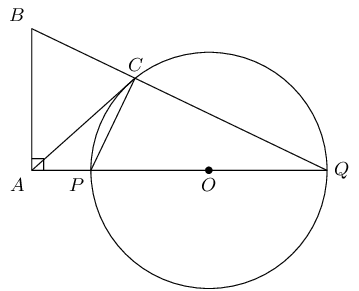Prove the following:

$$P\hat{C}Q = B\hat{A}P$$
$\begin{array}{rll} P\hat{C}Q &= \text{90}° & (\angle \text{ in semi-circle} ) \\ B\hat{A}Q &= \text{90}° & (\text{given } BA \perp AQ) \\ \therefore P\hat{C}Q &= B\hat{A}Q & \end{array}$

$$BAPC$$ is a cyclic quadrilateral

$\begin{array}{rll} P\hat{C}Q &= B\hat{A}Q & ( \text{proven} ) \\ \therefore &BAPC \text{ is a cyclic quad. } & ( \text{ext. angle = int. opp. } \angle ) \end{array}$
$$AB = AC$$
$\begin{array}{rll} C\hat{P}Q &= A\hat{B}C & ( \text{ext. } \angle \text{ of cyclic quad.}) \\ B\hat{C}P &= C\hat{P}Q + C\hat{Q}P & ( \text{ext. } \angle \text{ of } \triangle) \\ A\hat{C}P &= C\hat{Q}P & ( \text{tangent-chord} ) \\ \therefore B\hat{C}A &= C\hat{P}Q & \\ &= A\hat{B}C & \\ \therefore AB &= AC & (\angle \text{s opp. equal sides}) \end{array}$

$$TA$$ and $$TB$$ are tangents to the circle with centre $$O$$. $$C$$ is a point on the circumference and $$A\hat{T}B = x$$.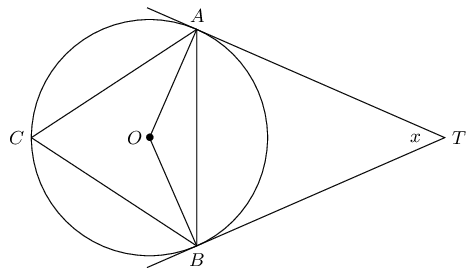Express the following in terms of $$x$$, giving reasons:

$$A\hat{B}T$$
$\begin{array}{rll} A\hat{B}T &= B\hat{A}T & (TA = TB) \\ &= \frac{\text{180}° - x}{2} & ( \text{sum } \angle \text{s of } \triangle TAB) \\ &= \text{90}° - \frac{x}{2} \end{array}$
$$O\hat{B}A$$
$\begin{array}{rll} O\hat{B}T &= \text{90}° & (\text{tangent } \perp \text{ radius}) \\ \therefore O\hat{B}A &= \text{90}° - \left( \text{90}° - \frac{x}{2} \right) & \\ &= \frac{x}{2} \end{array}$
$$\hat{C}$$
$\begin{array}{rll} \hat{C} &= A\hat{B}T & (\text{tangent chord}) \\ &= \text{90}° - \frac{x}{2} & \end{array}$